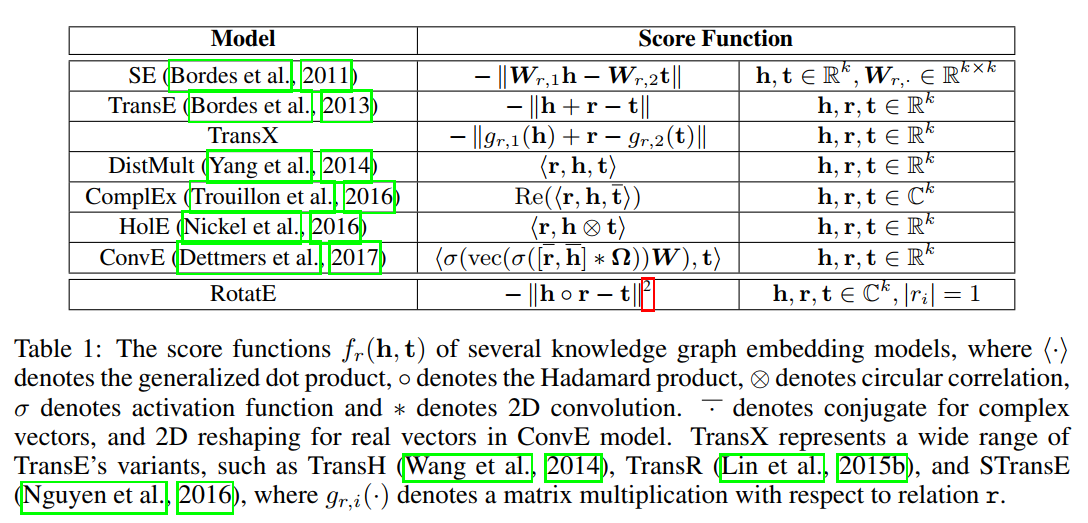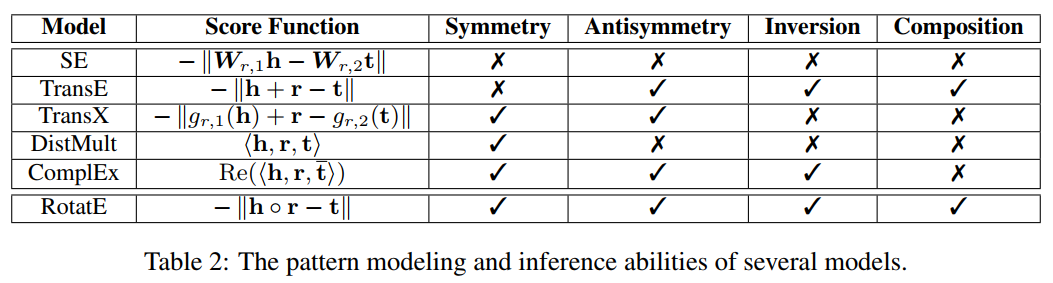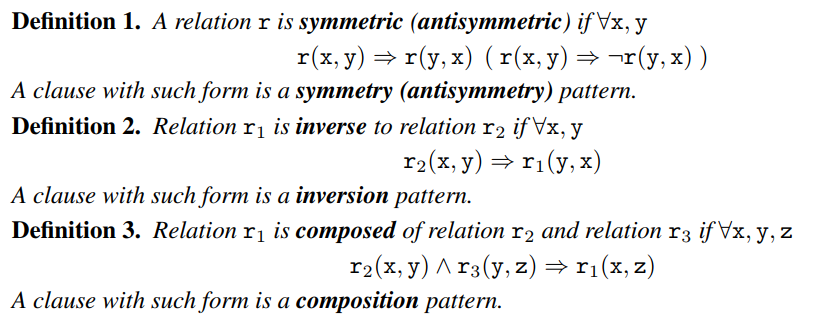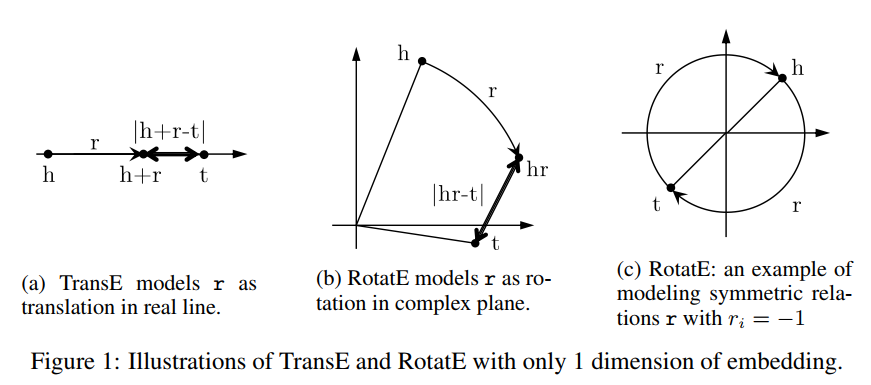# 图谱论文笔记3 - RotatE

Author: Steven Date: Jul 7, 2019 Updated On: May 5, 2022
Categories: KG
876 words in total, 3 minutes required.

RotatE模型将每个关系定义为从源实体到目标实体在复数向量空间的旋转。这篇文章也提出了一种自对抗的负采样技术。

• symmetry/antisymmetry （对称/非对称）： 如marriage是对称的；filiation（父子关系）是非对称的；
• inversion（倒置）：hypernym（上义词） and hyponym（下义词）；
• composition（组合）：母亲的丈夫是父亲；KGE模型对比KGE模型属性对比

RotatE的理论基础是欧拉恒等式，即

• $r$为symmetric，iff. 每个维度$r_i$满足$r_i = e^{0/i\pi} = \pm 1$；
• $r_1$和$r_2$互为inverse，iff. 二者的embedding互为共轭，$\mathbf{r}_2 = \bar{\mathbf{r}}_1$；
• $r_3 = e^{i\theta_3}$是$r_1 = e^{i\theta_1}$和$r_1 = e^{i\theta_2}$的composition； iff. $r_3 = r_1 \circ r_2$ (i.e., $\theta_3 = \theta_1 + \theta_2$)；

### RotatE建模

RotatE: RELATIONAL ROTATION IN COMPLEX VECTOR SPACERelation PatternsRotatE对对称性关系的建模

### RotatE优化

RotatE的loss function定义如下：